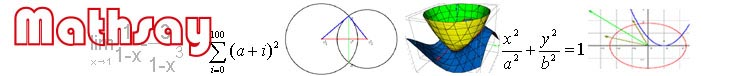Home

Support

Examples

Geometry

Trigonometry

Coordinate geometry

Function

Equation

Vector

3D geometry

3D plot

Limit & derivative

Math symbol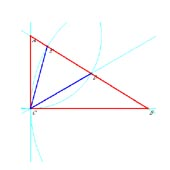Right triangle ABC, points D and E are in the side of BC. AD=AC and BE=AB. How much is angle DAE?In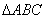,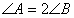and CD is of angle C. Prove CA+DA=BC.Rectangle ABCD, P is in the side of BC and Q is in the side of CD. PA intersects PQ in 45 degree. Prove BP+DQ=QP.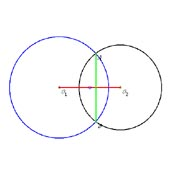Circles O1 and O2, their chord AB=8, calculate the distance between their centers (O1O2= ?).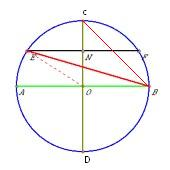In circle O, AB and CD are diameters, chord EF is vertical for OC and goes halves in OC. Prove.A triangle ABC, side AB=a, the height on side AB is h. Rectangle EFGH is inscribed, see image. How height is HE, the area of rectangle is maximum?  (Plot triangle ABC in setting AB=3 and its height = 3)